## Selina Concise Mathematics Class 10 ICSE Solutions Constructions (Circles)

Selina Publishers Concise Mathematics Class 10 ICSE Solutions Chapter 19 Constructions (Circles)

### Constructions Circles Exercise 19 – Selina Concise Mathematics Class 10 ICSE Solutions

Question 1.
Draw a circle of radius 3 cm. Mark a point P at a distance of 5 cm from the centre of the circle drawn. Draw two tangents PA and PB to the given circle and measure the length of each tangent.
Solution:
Steps of Construction: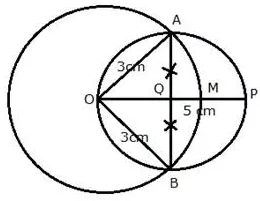1. Draw a circle with centre O and radius 3 cm.
2. From O, take a point P such that OP = 5 cm
3. Draw a bisector of OP which intersects OP at M.
4. With centre M, and radius OM, draw a circle which intersects the given circle at A and B.
5. Join AP and BP.
AP and BP are the required tangents.
On measuring AP = BP = 4 cm

Question 2.
Draw a circle of diameter of 9 cm. Mark a point at a distance of 7.5 cm from the centre of the circle. Draw tangents to the given circle from this exterior point. Measure the length of each tangent.
Solution:1. Draw a circle of diameter 9 cm, taking O as the centre.
2. Mark a point P outside the circle, such that PO = 7.5 cm.
3. Taking OP as the diameter, draw a circle such that it cuts the earlier circle at A and B.
4. Join PA and PB.
Thus, PA and PB are required tangents. PA = PB = 6 cm

Question 3.
Draw a circle of radius 5 cm. Draw two tangents to this circle so that the angle between the tangents is 45º.
Solution:
Steps of Construction:1. Draw a circle with centre O and radius BC = 5 cm
2. Draw arcs making an angle of 180º- 45º = 135º at O such that ∠AOB = 135º
3. AT A and B, draw two rays making an angle of 90º at each point which meet each other at point P, outside the circle.
4. AP and BP are the required tangents which make an angle of 45º with each other at P.

Question 4.
Draw a circle of radius 4.5 cm. Draw two tangents to this circle so that the angle between the tangents is 60º.
Solution:
Steps of Construction:1. Draw a circle with centre O and radius BC = 4.5 cm
2. Draw arcs making an angle of 180º – 60º = 120º at O such that ∠AOB = 120º
3. AT A and B, draw two rays making an angle of 90º at each point which meet each other at point P, outside the circle.
4. AP and BP are the required tangents which make an angle of 60º with each other at P.

Question 5.
Using ruler and compasses only, draw an equilateral triangle of side 4.5 cm and draw its circumscribed circle. Measure the radius of the circle.
Solution:
Steps of construction:1. Draw a line segment BC = 4.5 cm
2. With centers B and C, draw two arcs of radius 4.5 cm which intersect each other at A.
3. Join AC and AB.
4. Draw perpendicular bisectors of AC and BC intersecting each other at O.
5. With centre O, and radius OA or OB or OC draw a circle which will pass through A, B and C.
This is the required circumcircle of triangle ABC.
On measuring the radius OA = 2.6 cm

Question 6.
Using ruler and compasses only.
(i) Construct triangle ABC, having given BC = 7 cm, AB – AC = 1 cm and ∠ABC = 45°.
(ii) Inscribe a circle in the ∆ABC constructed in (i) above. Measure its radius.
Solution:
Steps of Construction:i) Construction of triangle:

• Draw a line segment BC = 7 cm
• At B, draw a ray BX making an angle of 45º and cut off BE = AB – AC = 1 cm
• Join EC and draw the perpendicular bisector of EC intersecting BX at A.
• Join AC.
∆ABC is the required triangle.

ii) Construction of incircle:

• Draw angle bisectors of ∠ABC and ∠ACB intersecting each other at O.
• From O, draw perpendiculars OL to BC.
• O as centre and OL as radius draw circle which touches the sides of the ∆ABC. This is the required in-circle of ∆ABC.
On measuring, radius OL = 1.8 cm

Question 7.
Using ruler and compasses only, draw an equilateral triangle of side 5 cm. Draw its inscribed circle. Measure the radius of the circle.
Solution:
Steps of Construction:1. Draw a line segment BC = 5 cm
2. With centers B and C, draw two arcs of 5 cm radius each which intersect each other at A.
3. Join AB and AC.
4. Draw angle bisectors of ∠B and ∠C intersecting each other at O.
5. From O, draw OL ⊥ BC.
6. Now with centre O and radius OL, draw a circle which will touch the sides of ∆ABC
On measuring, OL = 1.4 cm

Question 8.
Using ruler and compasses only,
(i) Construct a triangle ABC with the following data:
Base AB = 6 cm, BC = 6.2 cm and ∠CAB – 60°
(ii) In the same diagram, draw a circle which passes through the points A, B and C and mark its centre as O.
(iii) Draw a perpendicular from O to AB which meets AB in D.
(iv) Prove that AD = BD
Solution:
Steps of Construction:1. Draw a line segment AB = 6 cm
2. At A, draw a ray making an angle of 60º with BC.
3. With B as centre and radius = 6.2 cm draw an arc which intersects AX ray at C.
4. Join BC.
∆ABC is the required triangle.
5. Draw the perpendicular bisectors of AB and AC intersecting each other at O.
6. With centre O, and radius as OA or OB or OC, draw a circle which will pass through A, B and C.
7. From O, draw OD ⊥ AB.
Proof: In right ∆OAD and ∆OBD
OA = OB (radii of same circle)
Side OD = OD (common)

Question 9.
Using ruler and compasses only construct a triangle ABC in which BC = 4 cm, ∠ACB = 45° and perpendicular from A on BC is 2.5 cm. Draw a circle circumscribing the triangle ABC.
Solution:
Steps of Construction:1. Draw a line segment BC = 4 cm.
2. At C, draw a perpendicular line CX and from it, cut off CE = 2.5 cm.
3. From E, draw another perpendicular line EY.
4. From C, draw a ray making an angle of 45º with CB, which intersects EY at A.
5. Join AB.
∆ABC is the required triangle.
6. Draw perpendicular bisectors of sides AB and BC intersecting each other at O.
7. With centre O, and radius OB, draw a circle which will pass through A, B and C.
Measuring the radius OB = OC = OA = 2 cm

Question 10.
Perpendicular bisectors of the sides AB and AC of a triangle ABC meet at O.
(i) What do you call the point O?
(ii) What is the relation between the distances OA, OB and OC?
(iii) Does the perpendicular bisector of BC pass through O?
Solution:
Steps of Construction: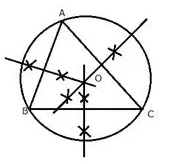1. O is called the circumcentre of circumcircle of ∆ABC.
2. OA, OB and OC are the radii of the circumcircle.
3. Yes, the perpendicular bisector of BC will pass through O.

Question 11.
The bisectors of angles A and B of a scalene triangle ABC meet at O.
i) What is the point O called?
ii) OR and OQ are drawn perpendiculars to AB and CA respectively. What is the relation between OR and OQ?
iii) What is the relation between angle ACO and angle BCO?
Solution:
Steps of Construction:1. O is called the incentre of the incircle of ∆ABC.
2. OR and OQ are the radii of the incircle and OR = OQ.
3. OC is the bisector of angle C
∴ ∠ACO = ∠BCO

Question 12.
i) Using ruler and compasses only, construct a triangle ABC in which AB = 8 cm, BC = 6 cm and CA = 5 cm.
ii) Find its incentre and mark it I.
iii) With I as centre, draw a circle which will cut off 2 cm chords from each side of the triangle.
Solution:
Steps of Construction:1. Draw a line segment BC = 6 cm.
2. With centre B and radius 8 cm draw an arc.
3. With centre C and radius 5 cm draw another arc which intersects the first arc at A.
4. Join AB and AC.
∆ABC is the required triangle.
5. Draw the angle bisectors of ∠B and ∠A intersecting each other at I. Then I is the incentre of the triangle ABC
6. Through I, draw ID ⊥ AB
7. Now from D, cut off $$D P=D Q=\frac{2}{2}=1 \mathrm{cm}$$
8. With centre I, and radius IP or IQ, draw a circle which will intersect each side of triangle ABC cutting chords of 2 cm each.

Question 13.
Construct an equilateral triangle ABC with side 6 cm. Draw a circle circumscribing the triangle ABC.
Solution:
Steps of Construction: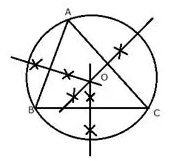1. Draw a line segment BC = 6 cm
2. With centers B and C, draw two arcs of radius 6 cm which intersect each other at A.
3. Join AC and AB.
4. Draw perpendicular bisectors of AC, AB and BC intersecting each other at O.
5. With centre O, and radius OA or OB or OC draw a circle which will pass through A, B and C.
This is the required circumcircle of triangle ABC.

Question 14.
Construct a circle, inscribing an equilateral triangle with side 5.6 cm.
Solution:
Steps of Construction: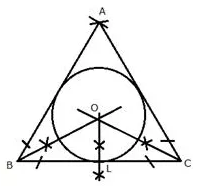1. Draw a line segment BC = 5.6 cm
2. With centers B and C, draw two arcs of 5.6 cm radius each which intersect each other at A.
3. Join AB and AC.
4. Draw angle bisectors of ∠B and ∠Cintersecting each other at O.
5. From O, draw OL ⊥ BC.
6. Now with centre O and radius OL, draw a circle which will touch the sides of ∆ABC
This is the required circle.

Question 15.
Draw a circle circumscribing a regular hexagon of side 5 cm.
Solution:
Steps of Construction: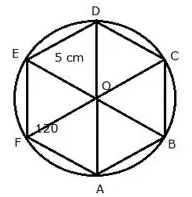1. Draw a regular hexagon ABCDEF with each side equal to 5 cm and each interior angle 120º.
2. Join its diagonals AD, BE and CF intersecting each other at O.
3. With centre as O and radius OA, draw a circle which will pass through the vertices A, B, C, D, E and F.
This is the required circumcircle.

Question 16.
Draw an inscribing circle of a regular hexagon of side 5.8 cm.
Solution:
Steps of Construction:1. Draw a line segment AB = 5.8 cm
2. At A and B, draw rays making an angle of 120° each and cut off AF = BC = 5.8 cm
3. Again F and C, draw rays making an angle of 120° each and cut off FE = CD = 5.8 cm.
4. Join DE. Then ABCDEF is the regular hexagon.
5. Draw the bisectors of ∠A and ∠B intersecting each other at O.
6. From O, draw OL ⊥ AB
7. With centre O and radius OL, draw a circle which touches the sides of the hexagon.
This is the required in circle of the hexagon.

Question 17.
Construct a regular hexagon of side 4 cm. Construct a circle circumscribing the hexagon.
Solution:
Steps of Construction: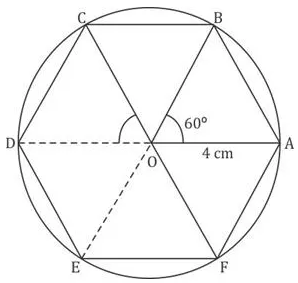1. Draw a circle of radius 4 cm with centre O
2. Since the interior angle of regular hexagon is 60o, draw radii OA and OB such that ∠AOB = 60°
3. Cut off arcs BC, CD, EF and each equal to arc AB on given circle
4. Join AB, BC, CD, DE, EF, FA to get required regular hexagon ABCDEF in a given circle.
The circle is the required circum circle, circumscribing the hexagon.

Question 18.
Draw a circle of radius 3.5 cm. Mark a point P outside the circle at a distance of 6 cm from the centre. Construct two tangents from P to the given circle. Measure and write down the length of one tangent.
Solution:
Steps of Construction: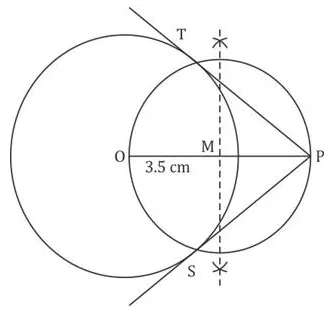1. Draw a line segment OP = 6 cm
2. With centre O and radius 3.5 cm, draw a circle
3. Draw the midpoint of OP
4. With centre M and diameter OP, draw a circle which intersect the circle at T and S
5. Join PT and PS.
PT and PS are the required tangents. On measuring the length of PT = PS = 4.8 cm

Question 19.
Construct a triangle ABC in which base BC = 5.5 cm, AB = 6 cm and m∠ABC =120˚.
i. Construct a circle circumscribing the triangle ABC.
ii. Draw a cyclic quadrilateral ABCD so that D is equidistant from B and C.
Solution:
Steps of Construction:
i.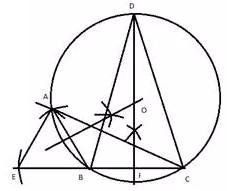a. Draw a line BC = 5.4 cm.
b. Draw AB = 6 cm, such that m ∠ABC = 120°.
c. Construct the perpendicular bisectors of AB and BC, such that they intersect at O.
d. Draw a circle with O as the radius.
ii.
(e) Extend the perpendicular bisector of BC, such that
it intersects the circle at D.
(f) Join BD and CD.
(g) Here BD = DC.

Question 20.
Using a ruler and compasses only :
(i) Construct a triangle ABC with the following data: AB = 3.5 cm, BC = 6 cm and ∠ABC = 120°.
(ii) In the same diagram, draw a circle with BC as diameter. Find a point P on the circumference of the circle which is equidistant from AB and BC.
(iii) Measure ∠BCP.
Solution:
Steps of constructions:

1. Draw a line segment BC = 6 cm.
At B, draw a ray BX making an angle of 120° with BC.
With B as centre and radius 3.5 cm, cut-off AB = 3.5 cm.
Join AC
Thus, ABC is the required triangle.

2. Draw perpendicular bisector MN of BC which cuts BC at point o.
With O as centre and radius = OB, draw a circle.
Draw angle bisector of ∠ABC which meets the cirde at point P.
Thus, point P is equidistant from AB and BC

3. On measuring, ∠BCP = 30°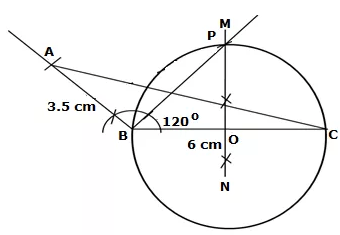Question 21.
Construct a ∆ABC with BC = 6.5 cm, AB = 5.5 cm, AC = 5 cm. Construct the incircle of the triangle. Measure and record the radius of the incircle.
Solution:
Steps of construction:

1. Draw BC = 6.5 cm.
2. With B as centre, draw an arc of radius 5.5 cm.
3. With C as oentre, draw an arc of radius 5 cm.
Let this arc meets the previous arc at A.
4. Join AB and AC to get ∆ABC.
5. Draw the bi sectors of ∠ABC and ∠ACB.
Let these bisectors meet each other at O.
6. Draw ON ⊥ BC.
7. With O as centre and radius ON, draw a inarcle that touches all the sides of ∆ABC
8. By measurement, radius ON = 1.5 cmQuestion 22.
Construct a triangle ABC with AB = 5.5 cm, AC = 6 cm and ∠BAC = 105°. Hence :
(i) Construct the locus of points equidistant from BA and BC.
(ii) Construct the locus of points equidistant from B and C.
(iii) Mark the point which satisfies the above two loci as P. Measure and write the length of PC.
Solution:
Steps of construction:

1. Draw AB = 5.5 cm
2. Construct ∠BAR = 1050
3. With centre A and radius 6 cm, aut off arc on AR at C.
4. Join BC. ABC is the required triangle.
5. Draw angle bisector BD of ∠ABC, which is the loss of points equidistant from BA and BC.
6. Draw perpendicular bisector EF of BC, which is the locus of points equidistant from B and C.
7. BD and EF intersect each other at point P.
Thus, P satisfies the above two lod.
By measurement, PC = 4.8 cm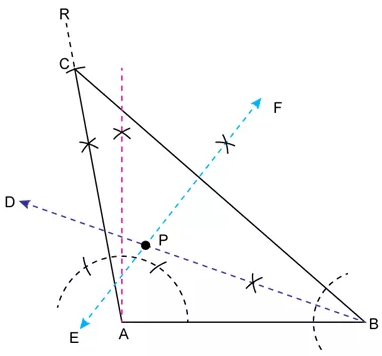Question 23.
Construct a regular hexagon of side 5 cm. Hence construct all its lines of symmetry and name them. (2016)
Solution:
Steps of construction:

1. Draw AF measuring 5 cm using a ruler.
2. With A as the centre and radius equal to AF, draw an arc above AF.
3. With F as the centre, and same radius cut the previous arc at Z
4. With Z as the centre, and same radius draw a circle passing through A and F.
5. With A as the centre and same radius, draw an arc to cut the circle above AF at B.
6. With B as the centre and same radius, draw an arc to cut the circle at C.
7. Repeat this process to get remaining vertices of the hexagon at D and E.
8. Join consecutive arcs on the circle to form the hexagon.
9. Draw the perpendicular bisectors of AF, FE and DE.
10. Extend the bisectors of AF, FE and DE to meet CD, BC and AB at X, L and O respectively.
11. Join AD, CF and EB.

These are the 6 lines of symmetry of the regular hexagon.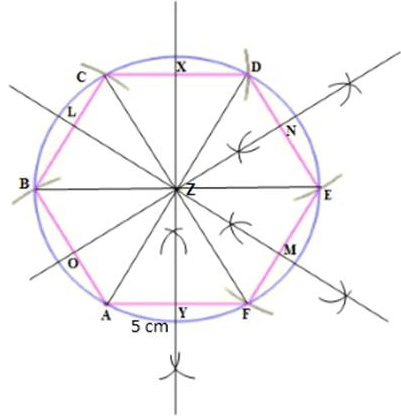Question 24.
Draw a line AB = 5 cm. Mark a point C on AB such that AC = 3 cm. Using a ruler and a compass only, construct:
(i) A circle of radius 2.5 cm, passing through A and C.
(ii) Construct two tangents to the circle from the external point B. Measure and record the length of the tangents.   (2016)
Solution:
Steps for construction:

1. Draw AB = 5 cm using a ruler.
2. With A as the centre cut an arc of 3 cm on AB to obtain C.
3. With A as the centre and radius 2.5 cm, draw an arc above AB.
4. With same radius, and C as the centre draw an arc to cut the previous arc and mark the intersection as O.
5. With O as the centre and radius 2.5 cm, draw a circle so that points A and C lie on the circle formed.
6. Join OB.
7. Draw the perpendicular bisector of OB to obtain the mid-point of OB, M.
8. With the M as the centre and radius equal to OM, draw a circle to cut the previous circle at points P and Q.
9. Join PB and QB. PB and QB are the required tangents to the given circle from exterior point B.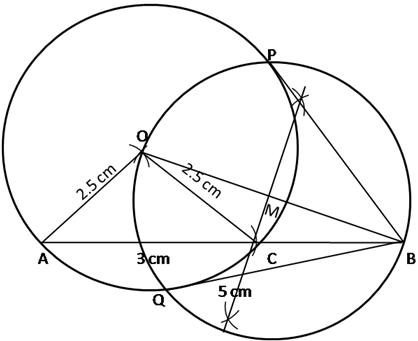QB = PB = 3 cm
That is, length of each tangent is 3 cm.

Solution 25.
Steps of construction :

1. Draw a line AB = 7 cm
2. Taking P as centre and same radius, draw an arc of a circle which intersects AB at M.
3. Taking M as centre and with the same radius as before drawn an arc intersecting previously drawn arc, at point N.
4. Draw the ray AX passing through N, then ∠XAB = 60°
5. Taking A as centre and radius equal to 5 cm, draw an arc cutting AX at C.
6. Join BC
7. The required triangle ABC is obtained.
8. Draw angle bisector of ∠CAB and ∠ABC
9. Mark their intersection as O
10. With O as center, draw a circle with radius ODSolution 26.
Steps for construction :

1. Draw BC = 6.8 cm.
2. Mark point D where BD = DC = 3.4 cm which is mid-point of BC.
3. Mark a point A which is intersection of arcs AD = 4.4 cm and AB = 5 cm from a point D and B respectively.
4. Join AB, AD and AC. ABC is the required triangle.
5. Draw bisectors of angle B and angle C which are ray BX and CY where I is the incentre of a circle.
6. Draw incircle of a triangle ABC.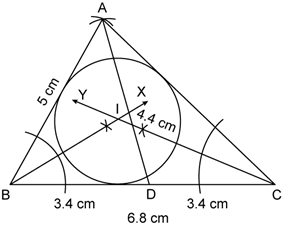Solution 27.
Steps for construction :

1. Draw concentric circles of radius 4 cm and 6 cm with centre of O.
2. Take point P on the outer circle.
3. Join OP.
4. Draw perpendicular bisectors of OP where M is the midpoint of OP.
5. Take a distance of a point O from the point M and mark arcs from M on the inner circle it cuts at point A and B respectively.
6. Join PA and PB.

We observe that PA and PB are tangents from outer circle to inner circle are equal of a length 4.5 cm each.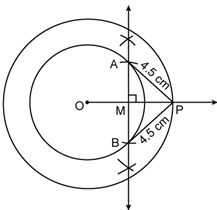Solution 28.
Steps for construction :

1. Draw BC = 7.2 cm.
2. Draw an angle ABC = 90°using compass.
3. Draw BD perpendicular to AC using compass.
4. Join BD.
5. Draw perpendicular bisectors of AB and BC which intersect at I, where I is the circumcentre of a circle.
6. Draw circumcircle using circumcentre I. we get radius of a circle is 4.7 cm.More Resources for Selina Concise Class 10 ICSE Solutions